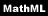#mml:math

\$Revision: 1666 \$

\$Date: 2002-06-12 07:19:37 -0400 (Wed, 12 Jun 2002) \$

mml:math — A MathML equation

## Synopsis

If the MathML Module is used, `Equation`s and `InlineEquation`s can include `mml:math` equations.

## Description

The Mathematical Markup Language Recommendation from the W3C defines the Mathematical Markup Language, or MathML. MathML is an XML application for describing mathematical notation and capturing both its structure and content.

A complete description of MathML is outside the scope of this reference.

## Examples

```<!DOCTYPE informalequation
PUBLIC "-//OASIS//DTD DocBook MathML Module V1.0//EN"
"http://www.oasis-open.org/docbook/xml/mathml/1.0/dbmathml.dtd">
<informalequation>
<mml:math><mml:apply><mml:divide/></mml:apply></mml:math>
</informalequation>
```